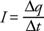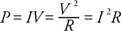## Current and Resistance

Electric circuits with charges in motion are commonplace in our technological society. Current, resistance, and electromotive force are concepts necessary to describe these circuits.

## Current

Current (I) is the amount of charge per time that passes through an area perpendicular to the flow:Current is measured in SI units of amperes (A), andThis definition for current can be applied to charges moving in a wire, in an electrolytic cell, or even in ionized gases.

In visualizing charge flowing through a circuit, it is not accurate to imagine the electrons moving very rapidly around the circuit. The average velocity, or drift velocity ( v b), of individual charges is low; the conduction electrons in a copper wire move on the order of 10 −4 m/s. The formula iswhere q is the charge on an electron, A is the cross‐sectional area of the wire, and n is the number of conduction electrons per cubic meter. At this rate, the time to travel 10 cm is about 11 minutes. It is obvious from experience that it does not take this long for a bulb to glow after the switch is closed. When the circuit is completed, the entire charge distribution responds almost immediately to the electric field and is set in motion almost simultaneously, even though individual charges move slowly.

The battery provides a voltage (V) between its terminals. The electric field set up in a wire connected to the battery terminals causes the current to flow, which occurs when the current has a complete conducting path from one terminal of the batter to the other—called a circuit. By convention, the direction of current in the external circuit (not in the battery) is the direction of motion of positive charges. In metals, the electrons are the moving charges, so the definition of the direction of current is opposite the actual flow of the negative charges in a wire. ( Note: Electric fields are not found in conductors with static charges as shown by Gauss's law, but electric fields can exist in a conductor when charges are in motion.)

The potential difference between the terminals of the battery when no current is present is called the electromotive force (emf). The historical term emf is a misnomer because it is measured in volts, not force units, but the terminology is still commonly used.

## Resistance and resistivity

Experimentally, it was found that current is proportional to voltage for conductors. The proportionality constant is the resistance in the circuit. This relationship is called Ohm's law: V = IR. Resistance is measured in ohms ( W): an ohm is equal to 1 volt/1 ampere.

The resistance of a conductor depends upon its length (l), its cross‐sectional area (A), and its resistivity ( r). The resistivity for a specific conductor can be found in a table of properties of materials. The unit of resistivity is the ohm‐meter. Resistance to current in a conductor arises because the flow of moving charges is impeded by the material of the wire. It is intuitive that the resistance should increase with the length of the wire, be inversely proportional to the cross‐sectional area (less resistance for a larger area), and depend upon the wire substance. The relationship between resistance and resistivity isNote: A resistor is a specific electronic component whose only function is to resist current. A resistance is generated by anything impeding current, for example, a light bulb or heating element.

## Electrical power and energy

Figure 1 shows a simple circuit of a battery with wires connecting it to a bulb. The filament in the bulb is a resistance shown in the circuit as R beside the symbol for a resistance. The symbol for the voltage of the battery is ε. Assume that the resistance in the connecting wires is negligible so that the light bulb is effectively the only resistance in the circuit. A constant potential difference is supplied by the battery—say, for example, 6 volts. When the current passes through the light bulb, charges move from a higher potential to a lower, with a difference of 6 volts. Energy is being converted into light and heat by the bulb filament.Figure 1

A simple circuit with a light bulb represented by the resistor R.

The rate of energy expenditure is power, given by any of the three expressions:Power is measured in units of watts (W):